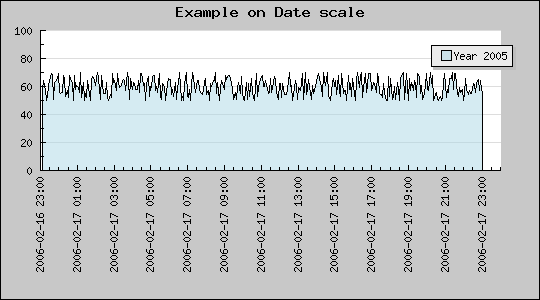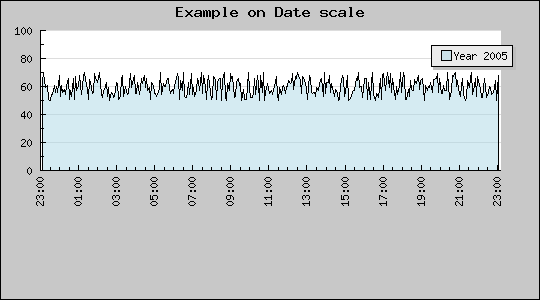Contents Previous Next

### 8.10.1 Using the automatic date/time scale

The easiest way to get a date time scale for the X-axis is to use the pre-defined "dat" scale. To be able to use that it is first necessary to include the module "jpgraph_date.php" and the specify the scale, for example as "datlin" in the call to SetScale() as the following code snippet shows.

```  require_once("../jpgraph.php"); require_once("../jpgraph_line.php"); require_once("../jpgraph_date.php"); ... \$graph  = new Graph(540, 300); \$graph->SetScale( 'datlin'); ...```

The above code snippet would create a date/linear scale for the graph. We will first show a basic use of the date scale and then go on to illustrate how it is possible to tailor the exact formatting of the date axis.

When using the fully automatic formatting the library will use the shortest possible string that uniquely identifieas a date/time. For example if the entire intervall falls within the same day only the time values will be displayed, if the intervall falls over several days then also the date will be added to the label.

As usual it is also possible to manually force a certain label formatting to be used this is illustrated below.

#### 8.10.1.1 Specifying the input data

The first basic example below creates a data set that emulates a sample rate every S second and falls over a total period of two days. It is important to note that the input data on the X-axis must be a in the form of timestamp data, i.e. the number of seconds from the system epoch. In PHP the current timestamp value is returned by the function time().

This means that it is always mandatory to specify two input vectors for a plot. For example to specify a line plot

```  \$line  = new LinePlot (\$data,\$xdata); ```

The example below show this in practiceFigure 76: Using the special date X-axis with all default values [src]

Please review the script (by following the [src] link) that creates this graph before continuing since we will base all further examples on the above example.

#### 8.10.1.2 Adjusting the start and end alignment

As can be seen from the above example the scale starts slightly before the first data point. Why? This is of course by purpose in order to make the first time label to start on an "even" value, in this case on an hour. Depending on the entire interval of the graph the start value will always be chosen to be the largest "even" time value, this could for example be on an even minute, even 30min, even hour, even day, even week and so on.

The alignment of the start (and end) vdate alignment can also be adjusted manually by using the two methods

• SetTimeAlign(\$aStartAlign,\$aEndAlign)
• SetDateAlign(\$aStartAlign,\$aEndAlign)

These method allow the alignment to be made by specifying one of the following constants.

For the SetTimeAlign() the following symbolic constants can be used

1. Alignment on seconds
• MINADJ_1, Align on a single second (This is the lowest resolution)
• MINADJ_5, Align on the nearest 5 seconds
• MINADJ_10, Align on the nearest 10 seconds
• MINADJ_15, Align on the nearest 15 seconds
• MINADJ_30, Align on the nearest 30 seconds
2. Alignment on minutes
• MINADJ_1, Align to the nearest minute
• MINADJ_5, Align on the nearest 5 minutes
• MINADJ_10, Align on the nearest 10 minutes
• MINADJ_15, Align on the nearest 15 minutes
• MINADJ_30, Align on the nearest 30 minutes
3. Alignment on hours
• HOURADJ_1, Align to the nearest hour
• HOURADJ_2, Align to the nearest two hour
• HOURADJ_3, Align to the nearest three hour
• HOURADJ_4, Align to the nearest four hour
• HOURADJ_6, Align to the nearest six hour
• HOURADJ_12, Align to the nearest tolw hour

For the SetDateAlign() the following symbolic constants can be used

1. Alignment on a day basis
• DAYADJ_1, Align on the start of a day
• DAYADJ_7, Align on the start of a week
2. Alignment on a monthly basis
• MONTHADJ_1, Align on a month start
• MONTHADJ_6, Align on the start of halfyear
3. Alignment on a yearly basis
• YEARADJ_1, Align on a year
• YEARADJ_2, Align on a bi-yearly basis
• YEARADJ_5, Align on a 5 year basis

Some examples will calrify the use of these methods.

Example 1. We want the time adjustment to start on an even quarter of an hour, i.e. an even 15 minute period.

```  \$graph ->xaxis->scale-> SetTimeAlign( MINADJ_15); ```

Example 2. We want the time to start on an even 2 hour

```  \$graph ->xaxis->scale-> SetTimeAlign( HOURADJ_2); ```

Example 3. We want the start to be on an even day

```  \$graph ->xaxis->scale-> SetDateAlign( DAYADJ_1); ```

#### 8.10.1.3 Adjusting the label format

The default label format always tries to use the shortest possible unique string. To manually set a manual scale the method SetDateFormat() is used for example as in

```  \$graph ->xaxis->scale-> SetDateFormat( 'H:i'); ```

The above example will force the labels to be displayed as hour (24h) and minutes.Figure 77: Using specific label format and align time axis to start and end on an even 10min [src]

#### 8.10.1.4 Adjusting the date scale density

As with the linear scale it is possible to indicate what density of scale ticks is needed. This is specified as usual with a call to Graph::SetTickDensity()

```  \$graph->SetTickDensity( TICKD_DENSE); ```

Contents Previous Next